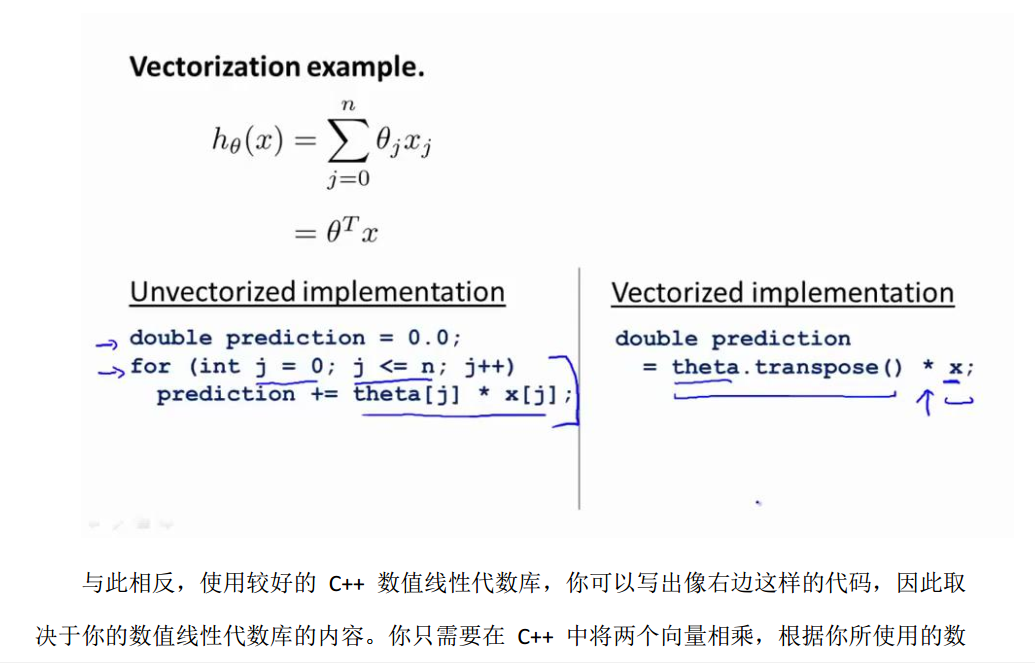# c++ vector(向量)一般使用方法的学习********正文1*********

vector(向量): C++中的一种数据结构,确切的说是一个类.它相当于一个动态的数组,当程序员无法知道自己需要的数组的规模多大时,用其来解决问题可以达到最大节约空间的目的.

1.用法:  首先在程序开头处加上#include<vector>以包含所需要的类文件vector

还有一定要加上using namespace std;

2.变量声明:

2.1 例:声明一个int向量以替代一维的数组:vector <int> a;(等于声明了一个int数组a[],大小没有指定,可以动态的向里面添加删除)。

2.2 例:用vector代替二维数组.其实只要声明一个一维数组向量即可,而一个数组的名字其实代表的是它的首地址,所以只要声明一个地址的向量即可,即:vector <int *> a.同理想用向量代替三维数组也是一样,vector <int**>a;再往上面依此类推.

3.函数用法

（1）a.assign(b.begin(), b.begin()+3); //b为向量，将b的0~2个元素构成的向量赋给a
（2）a.assign(4,2); //是a只含4个元素，且每个元素为2
（3）a.back(); //返回a的最后一个元素
（4）a.front(); //返回a的第一个元素
（5）a[i]; //返回a的第i个元素，当且仅当a[i]存在
（6）a.clear(); //清空a中的元素
（7）a.empty(); //判断a是否为空，空则返回ture,不空则返回false
（8）a.pop_back(); //删除a向量的最后一个元素
（9）a.erase(a.begin()+1,a.begin()+3); //删除a中第1个（从第0个算起）到第2个元素，也就是说删除的元素从a.begin()+1算起（包括它）一直到a.begin()+ 3（不包括它）
（10）a.push_back(5); //在a的最后一个向量后插入一个元素，其值为5

a.push_front(5);//在a的最前一个向量前插入一个元素，其值为5
（11）a.insert(a.begin()+1,5); //在a的第1个元素（从第0个算起）的位置插入数值5，如a为1,2,3,4，插入元素后为1,5,2,3,4
（12）a.insert(a.begin()+1,3,5); //在a的第1个元素（从第0个算起）的位置插入3个数，其值都为5
（13）a.insert(a.begin()+1,b+3,b+6); //b为数组，在a的第1个元素（从第0个算起）的位置插入b的第3个元素到第5个元素（不包括b+6），如b为1,2,3,4,5,9,8 ，插入元素后为1,4,5,9,2,3,4,5,9,8
（14）a.size(); //返回a中元素的个数；
（15）a.capacity(); //返回a在内存中总共可以容纳的元素个数
（16）a.rezize(10); //将a的现有元素个数调至10个，多则删，少则补，其值随机
（17）a.rezize(10,2); //将a的现有元素个数调至10个，多则删，少则补，其值为2
（18）a.reserve(100); //将a的容量（capacity）扩充至100，也就是说现在测试a.capacity();的时候返回值是100.这种操作只有在需要给a添加大量数据的时候才 显得有意义，因为这将避免内存多次容量扩充操作（当a的容量不足时电脑会自动扩容，当然这必然降低性能）
（19）a.swap(b); //b为向量，将a中的元素和b中的元素进行整体性交换
（20）a==b; //b为向量，向量的比较操作还有!=,>=,<=,>,<

（21） a.pop_front();//从容器的首部移走数据；

(22)   a.pop_back();//从容器的尾部移走数据；

# include<vector>
#include <iostream>
#include <algorithm>
using namespace std;
int main() {
//向量a中添加元素
vector<int> B; //创建了一个名字叫B的向量容器
for (int i = 0; i < 10; i++)
{
B.push_back(i);
cout << B[i]<< " ";
}
cout <<  '\n';

// 【误区】 要注意！！！虽然还不知道是否如此的解释这个错误
//int D = { 1,2,3,4,5,6 };
//vector<int> b;
//for (int i = 1; i <= 4; i++)
//{
//	b.push_back(D[i]);
//	//cout << b[i];  //出错哈，好像是下标只能用于获取已存在的元素，而现在的b[i]还是空的对象
//}
//for (int i = 0; i <= b.size() - 1; i++)
//	cout << b[i] << " ";

//也可以从数组中选择元素向向量中添加
int a = { 1,5,3,9,10,6 };
vector<int> b(a, a + 6);
cout << "向量：" ;
for (vector<int>::iterator it = b.begin(); it != b.end(); it++)
cout << *it << " ";
cout << '\n';

//将向量由小到大排序 1325,变成1235
sort(b.begin(),b.end());
cout << "排序的结果：";
for (vector<int>::iterator it = b.begin(); it != b.end(); it++)
{
cout << *it << " ";
}
cout << '\n';

     //将向量前后倒置，1234，变成4321
reverse(b.begin(), b.end());cout << "倒置的结果：";for (vector<int>::iterator it = b.begin(); it != b.end(); it++){cout << *it << " " ;}cout << '\n';
     //将向量b复制到B中去，从0序开始复制，B后面的数不变
copy(b.begin(), b.end(), B.begin());

cout << "把b复制到B的前6位的结果：" << '\n';
for (int i = 0; i <= B.size() - 1; i++) //通过下标方式从向量中读取元素{cout << B[i] << " " ;}cout << '\n';vector<int>::iterator found; //是定义向量迭代器found = find(B.begin(), B.end(), 1);cout << "寻找在b中等于10的结果："; if (found != B.end()) // finded{std::cout << "The element is found:";std::cout << *found << '\n';}system("pause");}

（1）sort(a.begin(),a.end()); //对a中的从a.begin()（包括它）到a.end()（不包括它）的元素进行从小到大排列
（2）reverse(a.begin(),a.end()); //对a中的从a.begin()（包括它）到a.end()（不包括它）的元素倒置，但不排列，如a中元素为1,3,2,4,倒置后为4,2,3,1
（3）copy(a.begin(),a.end(),b.begin()+1); //把a中的从a.begin()（包括它）到a.end()（不包括它）的元素复制到b中，从b.begin()+1的位置（包括它）开始复制，覆盖掉原有元素

（4）find(a.begin(),a.end(),10); //在a中的从a.begin()（包括它）到a.end()（不包括它）的元素中查找10

********正文2*********

// find example
#include <iostream>     // std::cout
#include <algorithm>    // std::find
#include <vector>       // std::vector

int main () {
// using std::find with array and pointer:
int myints[] = { 10, 20, 30, 40 };
int * p;

p = std::find (myints, myints+4, 30);
if (p != myints+4)
std::cout << "Element found in myints: " << *p << '\n';
else
std::cout << "Element not found in myints\n";

// using std::find with vector and iterator:
std::vector<int> myvector (myints,myints+4);
std::vector<int>::iterator it;

it = find (myvector.begin(), myvector.end(), 30);
if (it != myvector.end())
std::cout << "Element found in myvector: " << *it << '\n';
else
std::cout << "Element not found in myvector\n";

return 0;
}
Output:
Element found in myints: 30
Element found in myvector: 30


********正文3*********

vector<int>::iterator是什么意思？

vector<int>是声明向量容器
例如 verctor<int> v,就是创建了一个名字叫v的向量容器。
vector<int>::iterator是定义向量迭代器
例如，vector<int>::iterator it  就是定义了一个名字叫it 的向量迭代器
for(it=v.begin();it!=v.end();it++)
cout<<*it<<endl;

                                                                                *********总结 开始*****
vector<int> 是向量类型，而vector<int>::iterator是向量的迭代器类型，vector<int>::iterator用于迭代vector<int>中的int数据
                                                                                 *********总结 结束*****